# Converting Units Of Area And Volume Worksheet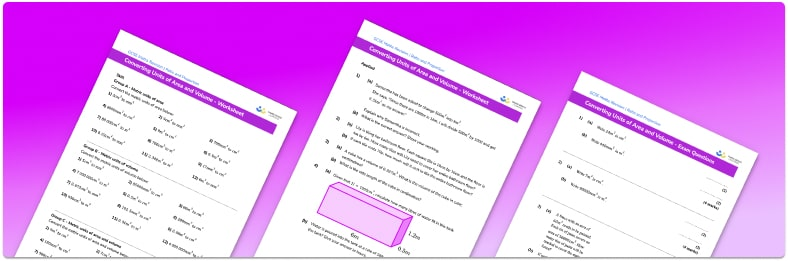Help your students prepare for their Maths GCSE with this free converting units of area and volume worksheet of 34 questions and answers

• Section 1 of the converting units of area and volume worksheet  contains 27 skills-based converting units of area and volume questions, in 3 groups to support differentiation
• Section 2 contains 3 applied converting units of area and volume questions with a mix of worded problems and deeper problem solving questions
• Section 3 contains 4 foundation and higher level GCSE exam style questions on converting units of area and volume
• Answers and a mark scheme for all converting units of area and volume questions are provided
• Questions follow variation theory with plenty of opportunities for students to work independently at their own level
• All questions created by fully qualified expert secondary maths teachers

Suitable for GCSE maths revision for AQA, OCR and Edexcel exam boards

• This field is for validation purposes and should be left unchanged.

You can unsubscribe at any time (each email we send will contain an easy way to unsubscribe). To find out more about how we use your data, see our privacy policy.

### Converting units of area and volume at a glance

Converting metric units of area and volume is an extension of converting metric units of length. Converting between different units, to smaller units or larger units, might be done to make a measurement easier to understand or to perform calculations.

When converting between metric units of area, we need to square the conversion rate for the units of length. For example, there are 10 millimetres in a centimetre so to convert from centimetres to millimetres we multiply by 10 . To convert from centimetres squared to millimetres squared we multiply by 10^2 . The reason for this is that we are working in two dimensions and 1cm^2 = 1cm \times 1cm = 10mm \times 10mm = 100mm^2 .

Similarly, when converting between metric units of volume, we need to cube the conversion rate for the units of length. For example, to convert between km and m we multiply by 1000 since there are 1000m in 1km . Working in three dimensions,

1km^3 = 1km \times 1km \times 1km = 1000m \times 1000m \times 1000m = 1 000 000 000m^3 .

Looking forward, students can then progress to additional conversion worksheets and other ratio and proportion worksheets, for example a ratio worksheet or a simplifying and equivalent ratios worksheet.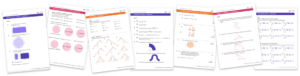For more teaching and learning support on Ratio and Proportion our GCSE maths lessons provide step by step support for all GCSE maths concepts.

## Related worksheets

Unit Conversion Worksheet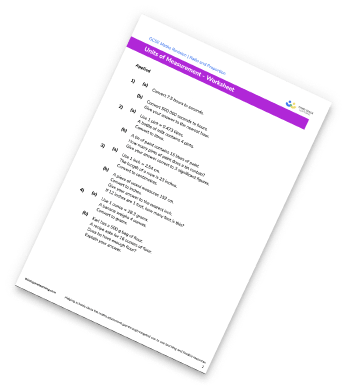Converting Units Of Measure Worksheet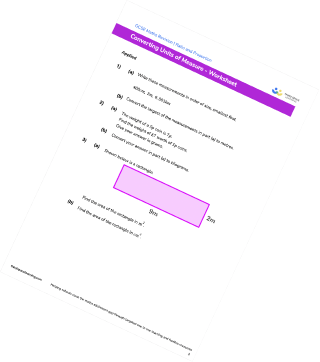Metric Conversion Worksheet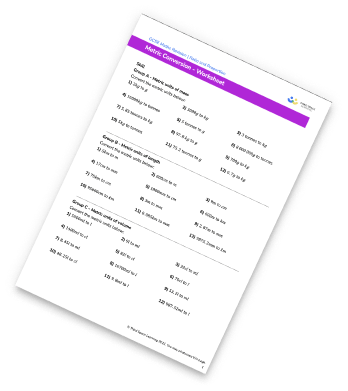Time Unit Conversion Worksheet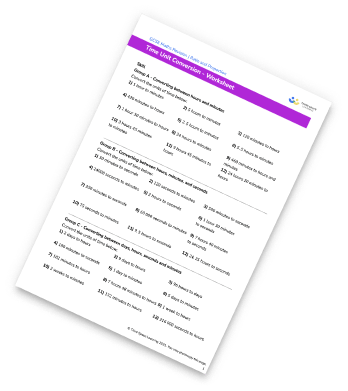## Do you have KS4 students who need more focused attention to succeed at GCSE?There will be students in your class who require individual attention to help them succeed in their maths GCSEs. In a class of 30, it’s not always easy to provide.

Help your students feel confident with exam-style questions and the strategies they’ll need to answer them correctly with our dedicated GCSE maths revision programme.

Lessons are selected to provide support where each student needs it most, and specially-trained GCSE maths tutors adapt the pitch and pace of each lesson. This ensures a personalised revision programme that raises grades and boosts confidence.

Find out more